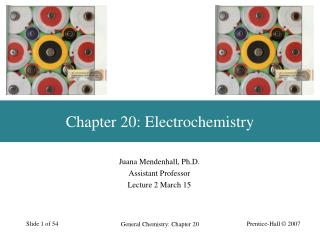# Chapter 20: Electrochemistry - PowerPoint PPT PresentationDownload PresentationChapter 20: Electrochemistry

Chapter 20: ElectrochemistryDownload Presentation## Chapter 20: Electrochemistry

- - - - - - - - - - - - - - - - - - - - - - - - - - - E N D - - - - - - - - - - - - - - - - - - - - - - - - - - -
##### Presentation Transcript

1. Chapter 20: Electrochemistry Juana Mendenhall, Ph.D. Assistant Professor Lecture 2 March 15 General Chemistry: Chapter 20

2. Objectives • Describe and illustrate how a voltaic cell operates, with the concepts of electrodes, salt bridges, half-cell equations, net cell reaction and cell diagram. • Describe the standard hydrogen electrode and explain how other standard electrode potentials are related to it. • Using tabulated standard potentials, Eº, to determine E°cell for an oxidation-reduction reaction and predict whether the reaction is spontaneous. General Chemistry: Chapter 20

3. An Electrochemical Half Cell Anode Cathode General Chemistry: Chapter 20

4. An Electrochemical Cell General Chemistry: Chapter 20

5. Terminology • Electromotive force, Ecell. • The cell voltage or cell potential. • Cell diagram. • Shows the components of the cell in a symbolic way. • Anode (where oxidation occurs) on the left. • Cathode (where reduction occurs) on the right. • Boundary between phases shown by |. • Boundary between half cells (usually a salt bridge) shown by ||. General Chemistry: Chapter 20

6. Terminology Zn(s) | Zn2+(aq) || Cu2+(aq) | Cu(s) Ecell = 1.103 V This typical electrochemical cell was one of the first developed to produce electrical energy (to run Morse code machines). It is called a Daniell cell. General Chemistry: Chapter 20

7. Terminology • Galvanic cells. • Produce electricity as a result of spontaneous reactions. • Electrolytic cells. • Non-spontaneous chemical change driven by electricity. • Couple, M|Mn+ • A pair of species related by a change in number of e-. General Chemistry: Chapter 20

8. Example A galvanic cell consists of a Mg electrode in a 1.0M Mg(NO3)2 solution and a Ag electrode in a 1.0M AgNO3 solution. Calculate the standard emf of this electrochemical cell at 25º C. Mg2+(aq) + 2e- Mg(s) Eº = -2.37 V Answer Ag+(aq) + e- Ag(s) °E = 0.80 V Ag+ will oxidize the Mg2+ (diagonal rule): Anode: Mg(s) Mg2+(aq) + 2e- Cathode: 2Ag+(aq) + 2e- 2Ag(s) Overall: Mg (s) + 2Ag+(aq) Mg2+(aq)+ 2Ag(s) General Chemistry: Chapter 20

9. Example cont. Eº = Eºmg/mg2+ + EºAg+/Ag = 2.37 V + 0.80 V = 3.17 V The sign of the emf of the cell allows us to predict the spontaneity of a redox rxn. Recall that under steady-state conditions for rxns & products, the redox rxn is spontaneous in the forward direction if the standard emf of the cell is positive. If it is negative, Eºcell does not mean the rxn will not occur, it means the the equilibrium will shift to the left. General Chemistry: Chapter 20

10. Standard Electrode Potentials • Cell voltages, the potential differences between electrodes, are among the most precise scientific measurements. • The potential of an individual electrode is difficult to establish. • Arbitrary zero is chosen. The Standard Hydrogen Electrode (SHE) General Chemistry: Chapter 20

11. Standard Hydrogen Electrode 2 H+(a = 1) + 2 e- H2(g, 1 bar) E° = 0 V Pt|H2(g, 1 bar)|H+(a = 1) General Chemistry: Chapter 20

12. Standard Electrode Potential, E° • E° defined by international agreement. • The tendency for a reduction process to occur at an electrode. • All ionic species present at a=1 (approximately 1 M). • All gases are at 1 bar (approximately 1 atm). • Where no metallic substance is indicated, the potential is established on an inert metallic electrode (ex. Pt). General Chemistry: Chapter 20

13. Reduction Couples Cu2+(1M) + 2 e-→ Cu(s) E°Cu2+/Cu = ? Pt|H2(g, 1 bar)|H+(a = 1) || Cu2+(1 M)|Cu(s) E°cell = 0.340 V anode cathode Standard cell potential: the potential difference of a cell formed from two standard electrodes. E°cell = E°cathode -E°anode General Chemistry: Chapter 20

14. Standard Cell Potential Pt|H2(g, 1 bar)|H+(a = 1) || Cu2+(1 M)|Cu(s) E°cell = 0.340 V E°cell = E°cathode -E°anode E°cell = E°Cu2+/Cu -E°H+/H2 0.340 V = E°Cu2+/Cu -0 V E°Cu2+/Cu = +0.340 V H2(g, 1 atm) + Cu2+(1 M) → H+(1 M) + Cu(s) E°cell = 0.340 V General Chemistry: Chapter 20

15. Ecell, ΔG, and Keq • Cells do electrical work. • Moving electric charge. • Faraday constant, F = 96,485 C mol-1 elec = -nFE Michael Faraday 1791-1867 ΔG = -nFE ΔG° = -nFE° General Chemistry: Chapter 20

16. Example Calculate the standard free-energy for the following reaction @ 25ºC: 2Au(s) + 3Ca2+ (aq) 2Au3+(aq) +3Ca(s) Answer: First we break up the overall rxn into half-rxns: Oxidation: 2Au(s) 2Au3+(aq) + 6e- Reduction: 3Ca2+ (aq) + 6e- +3Ca(s) Eº = EºAu/Au3+ + EºCa2+/Ca = -1.50 V - 2.87 V = -4.37 V Gº = -nFEº = - (6 mol)(96,500 J/V*mol)(-4.37 V) = 2.53 x 103 kJ General Chemistry: Chapter 20

17. Spontaneous Change • ΔG < 0 for spontaneous change. • Therefore E°cell > 0 because ΔGcell = -nFE°cell • E°cell > 0 • Reaction proceeds spontaneously as written. • E°cell = 0 • Reaction is at equilibrium. • E°cell < 0 • Reaction proceeds in the reverse direction spontaneously. General Chemistry: Chapter 20

18. Combining Half Reactions Fe3+(aq) + 3e-→ Fe(s) E°Fe3+/Fe = ? Fe2+(aq) + 2e-→ Fe(s) E°Fe2+/Fe = -0.440 V ΔG°= +0.880 J Fe3+(aq) + 3e-→ Fe2+(aq) E°Fe3+/Fe2+ = 0.771 V ΔG°= -0.771 J Fe3+(aq) + 3e-→ Fe(s) E°Fe3+/Fe = +0.331 V ΔG°= +0.109 V ΔG°= +0.109 V = -nFE° E°Fe3+/Fe= +0.109 V /(-3F) = -0.0363 V General Chemistry: Chapter 20